# Draw rough diagrams of two angles which have (a) One point common (b) Two points in common (c) Three points in common (d) Four points in common (e) One ray in common.

Solutions:

(a) O is a common point between ∠COD and ∠AOB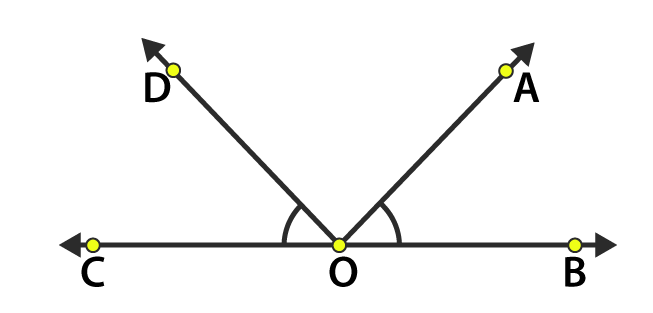(b) O and B are common points between ∠AOB and ∠BOC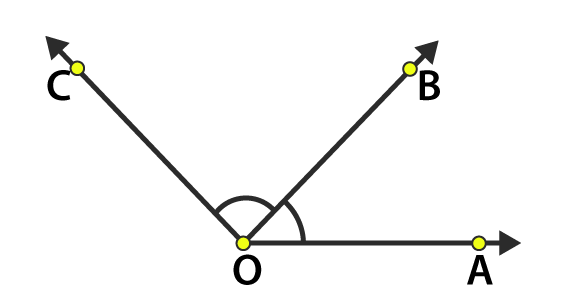(c) O, E and B are common points between ∠AOB and ∠BOC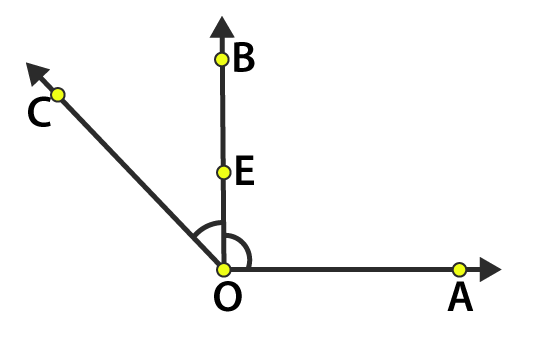(d) O, E, D and A are common points between ∠BOA and ∠COA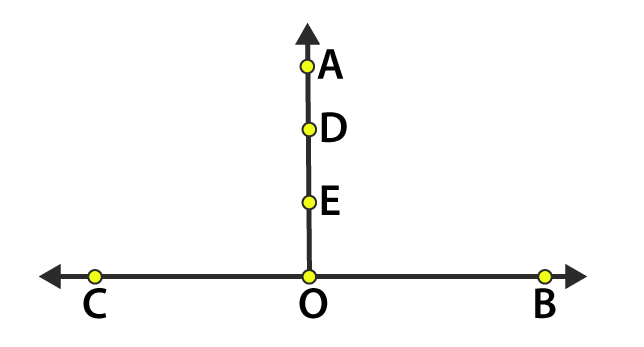(e) OC is common ray between ∠BOC and ∠AOC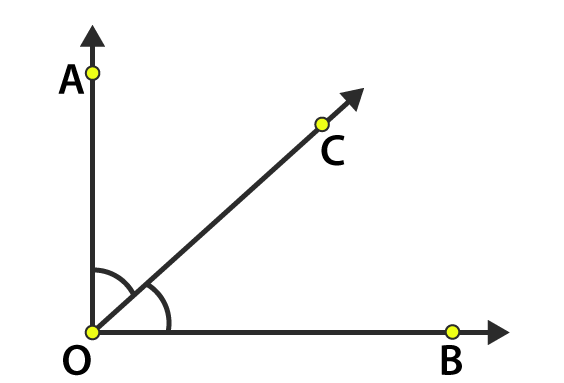(94)(22)